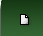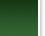COMPUTER GRAPHICSA.A. Ivanov On Efficient Schemes of Estimating the Degree of Manipulability of Aggregation ProceduresIMAGE PROCESSING METHODSCONTROL SYSTEMSПРИКЛАДНЫЕ АСПЕКТЫ ИНФОРМАТИКИA.A. Ivanov On Efficient Schemes of Estimating the Degree of Manipulability of Aggregation ProceduresAbstract. We study the algorithms for evaluation of manipulatility of aggregation procedures. We consider 27 known aggregation procedures and generalized scoring rules, when the weights of second or second and third alternatives are different. Both Impartial Culture and Impartial Anonymous Culture are studied. We calculate 6 manipulability indices including well-known Nitzan-Kelly index. We provide asymptotic estimations of the computational complexity. It is shown that optimization allows to calculate manipulability indices for aggregation procedures for the number of agents between 3 and 100 and for 3, 4 and 5 alternatives. The algorithms and their structures are discussed. Keywords: Manipulation, manipulability indices, aggregation procedures, scoring social choice rules. PP. 38-50. DOI 10.14357/20718632200204 References1. Gibbard A. Manipulation of voting schemes // Econometrica. 1973. Vol. 41. Р. 587–601.2. Satterthwaite M. Strategy-proofness and Arrow's conditions: existence and correspondence theorems for voting procedures and social welfare functions // Journal of Economic Theory. 1975. Vol. 10. Р. 187–217.3. Chamberlin J. R. An investigation into the relative manipulability of four voting systems // Behavioral Science. 1985. Vol.30(4). P.195–203.4. Aleskerov F., Kurbanov E. Degree of manipulability of social choice procedures // Alkan А. et al. (eds.) Current Trends in Economics. Studies in Economic Theory. Berlin Heidelberg, N.Y.: Springer, 1999. Vol.8. P.13-27.5. Aleskerov F., Karabekyan D., Sanver R., Yakuba V. On manipulability of positional voting rules // SERIEs: Journal of the Spanish Economic Association. 2011. Vol. 2 (4). P. 431-446.6. Aleskerov F., Karabekyan D., Sanver R., Yakuba V. On the manipulability of voting rules: Case of 4 and 5 Alternatives // Mathematical Social Sciences. 2012. Vol. 64 (1). P. 67–73.7. Pritchard G., Wilson M. Exact results on manipulability of positional voting rules// Social Choice and Welfare. 2007. Vol. 29. Р. 487–513.8. Favardin P., Lepelley D. Some further results on the manipulability of social choice rules // Social Choice and Welfare. 2006. Vol. 26. Р. 485–509.9. Nitzan S. The vulnerability of point-voting schemes to preference variation and strategic manipulation // Public Choice. 1985. Vol. 47. P. 349–370.10. Kelly J. Almost all social choice rules are highly manipulable, but few aren't // Social Choice and Welfare. 1993. Vol. 10. Р. 161–175.11. Xia, L., Conitzer, V. Generalized scoring rules and the frequency of coalitional manipulability // Proceedings of the 9th ACM conference on Electronic commerce. 2008, P. 109-118.12. Karabekyan, D.S., «Manipulation in collective decision making», Candidate of Science Thesis, Moscow, 2012/2021 / 03 2021 / 02 2021 / 01 2020 / 04© ФИЦ ИУ РАН 2008-2018. Создание сайта "РосИнтернет технологии".